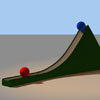#### You may also like### Lunar Leaper

Gravity on the Moon is about 1/6th that on the Earth. A pole-vaulter 2 metres tall can clear a 5 metres pole on the Earth. How high a pole could he clear on the Moon?### Which Twin Is Older?

A simplified account of special relativity and the twins paradox.### Whoosh

A ball whooshes down a slide and hits another ball which flies off the slide horizontally as a projectile. How far does it go?

# The Lorentz Force Law

##### Age 16 to 18Challenge Level

In Cartesian coordinates, the position ${\bf r}$ of the charged particle at time $t$ is determined by three functions$x(t), y(t), z(t)$, so that $${\bf r} = (x(t), y(t), z(t))$$

In component form, the Lorentz force law becomes

$$\left(\frac{m}{q} \ddot{x}, \frac{m}{q} \ddot{y}, \frac{m}{q} \ddot{z}\right) = \left( E_x+\dot{y}B_z-\dot{z}B_y, E_y+\dot{z}B_x-\dot{x}B_z, E_z+\dot{x}B_y-\dot{y}B_x \right)$$

You can then equate components to make three separate (simultaneous) differential equations.

Try differentiating these to solve for particular cases of ${\bf E}$ and ${\bf B}$.

You can make life easier for yourself by supposing the the electric and magnetic fields do not change over time.